Are you more of a visual learner? Check out our online video lectures and start your calculus course now for free!Image: “statistics” by geralt. License: CC0 1.0

## Introduction

Inferences for a population mean are made using a sample mean when the population mean is unknown. There are three ways to make inferences related to a population mean:

1. Point estimate
2. Interval estimate
3. Hypothesis testing

## Point Estimation

Point estimation involves making inferences about the population mean using a single number, such as the sample mean and sampling distribution mean. The single value is calculated from the sample.

### Sample mean

A sample is a small portion meant to represent the whole. The simplest way to make an inference about the population mean is to draw a small sample out of the population, compute its mean, and use it as an estimate of the population.

Example: suppose you want to estimate the average age of the people who suffer from cancer. It would be very costly and time-consuming to contact every person on the planet who is suffering from cancer; thus, a sample mean would work. You can contact 30 cancer patients and compute the mean of their ages and use it as an estimation of the population mean. This is cost-effective and less time-consuming, but it is not very accurate.

### Sampling distribution

This is a more sophisticated method than calculating a single sample mean. It involves drawing various samples from the population (as the name “sampling distribution” suggests), computing the mean of each sample, and then computing the mean of the means of each sample. The key to this method is that the samples must be drawn from the population in a random manner. This would be a more accurate point estimate of the population mean. This improves the chances of accuracy, especially if the sample selection is not biased.

Example: suppose we draw five random samples from the population of cancer patients, compute the mean of each sample, and finally compute the mean of the five means. This is shown as follows:

 Sample 1 Sample 2 Sample 3 Sample 4 Sample 5 10 80 40 80 10 10 80 40 80 10 20 10 40 10 20 30 10 40 10 30 10 10 30 10 20 80 0 30 10 80 0 20 40 10 0 10 30 40 10 20 50 70 0 70 50 Mean = 24 34 33 32 27 Mean of sampling distribution = 24 + 34 + 33 + 32 + 27 / 5 = 30

The mean of the sampling distribution was 30; thus, we can conclude that the average age of cancer patients is 30 years.

## Interval Estimation

Interval estimation involves making inferences about the population mean using a range of values, such as the confidence interval. Confidence interval estimation is the range of likely values of the parameter with a specified level of confidence. For instance, a 95% confidence interval would mean that if we were to take 100 different samples and calculate a 95% confidence interval, then 95 of 100 would contain the true mean value. The confidence interval under various scenarios is calculated as follows:

### Confidence interval when population standard deviation is known

When the population standard deviation is known, we calculate the confidence interval as follows: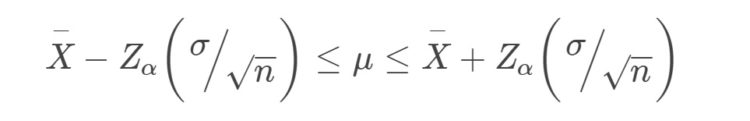In this formula, x bar is the sample mean, ơ is population standard deviation, n is the sample size, and z is 1.645 for 90% confidence, 2 for 95% confidence, and 2.576 for 99% confidence.

For example, suppose that the sample mean is 1.8, the population standard deviation is 0.5, and the sample size is 36. The confidence interval would be:

• (1.8 + 1.645 * (0.5 / √ 36), 1.8 – 1.645 * (0.5 / √ 36)) → (1.9370, 1.6629) at 90% confidence. That is, we can be sure 90% of the time that the ‘population mean’ would lie in the range 1.6629 to 1.9370.
• (1.8 + 2 * (0.5 / √ 36), 1.8 – 2 * (0.5 / √ 36)) → (1.9667, 1.6333) at 95% confidence. That is, we can be sure 95% of the time that the ‘population mean’ would lie in the range of 1.9667 to 1.6333.
• (1.8 + 2.576 * (0.5 / √ 36), 1.8 – 2.576 * (0.5 / √ 36)) → (2.0146, 1.5853) at 99% confidence. That is, we can be sure 99% of the time that the ‘population mean’ would lie in the range 1.5853 to 2.0146.

### Confidence interval when population standard deviation is unknown

When the population standard deviation is unknown, we calculate the confidence interval using the sample standard deviation: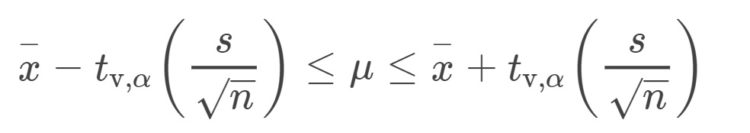In this formula, x bar is the sample mean, s is the sample standard deviation, n is the sample size, and t depends on the degrees of freedom (i.e., n-1). The t-distribution is as follows: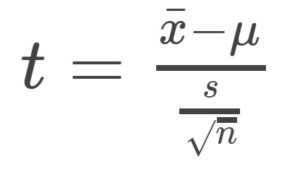For example, suppose that the sample mean is 1.8, the sample standard deviation is 1.3, and the sample size is 36. The confidence interval would be:

• For 90% confidence, the interval would be: (1.8 + 1.697 * (1.3 / √ 36), 1.8 – 1.697 * (1.3 /    √ 36)) → (2.1676, 1.4323). That is, we can be sure 90% of the time that the ‘population mean’ would lie in the range 1.4323 to 2.1676.
• For 95% confidence, the interval would be: (1.8 + 2.042 * (1.3 / √ 36), 1.8 – 2.042 * (1.3 /    √ 36)) → (2.2424, 1.3575). That is, we can be sure 90% of the time that the ‘population mean’ would lie in the range 1.3575 to 2.2424.
• For 99% confidence, the interval would be: (1.8 + 2.750 * (1.3 / √ 36), 1.8 – 2.750 * (1.3 /    √ 36)) → (2.3958, 1.2041). That is, we can be sure 90% of the time that the ‘population mean’ would lie in the range 1.2041 to 2.3958.

Limitations
We cannot use confidence intervals when the sample size is small (i.e., less than 30) and when the population is not normally distributed.

## Hypothesis testing

Hypothesis testing involves making inferences about the population mean by statistically testing certain claims about the population mean, such as the population mean is equal to x, or less than x, or greater than x. Study statistics estimating population parameters are the basis of developing a hypothesis since the true values of populations are difficult to determine.

### Hypothesis testing µ ≠ x

The first type of hypothesis testing related to the population mean is to test whether the population mean is equal to a certain value x. This is also referred to as the two-tailed test. The steps for conducting such a hypothesis test are as follows:

Step 1: Stating the hypothesis

H0: Null Hypothesis: µ = 20: the population mean is equal to 20.
HA: Alternative Hypothesis: µ ≠ 20: the population mean is not equal to 20.

Step 2: Computing the test statistic

The test statistic is calculated as follows: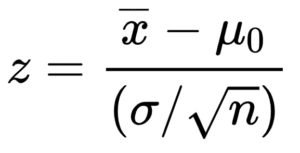In this formula, x bar is the sample mean, µo is the hypothesized mean, ơ is the population standard deviation, and n is the sample size. Suppose the sample mean is 24, the population standard deviation is 9.14 and the sample size is 64, then the z-statistic would be equal to = (24 – 20) / (9.14 / √ 64) = 4 / (9.14/8) = 3.5.

Step 3: Determining the critical values/rejection region

There are various rejection regions depending upon the level of significance (α).

 Two-tailed test α Reject Ho if Z statistic is: 0.20 Less than -1.282 OR more than +1.282 0.10 Less than -1.645 OR more than +1.645 0.05 Less than -1.96 OR more than +1.96 0.010 Less than -2.576 OR more than +2.576 0.001 Less than -3.291 OR more than +3.291 0.0001 Less than -3.819 OR more than +3.819

Step 4: Make a conclusion

The z statistic in the example is +3.5. This is higher than +3.291, so we can reject the Ho at the 0.1% significance level, but it is not higher than +3.819, so we cannot reject H0 at 0.01%. Finally, we conclude that we have enough evidence to reject the claim that the population mean is equal to 20.

### Hypothesis testing µ > x

The second type of hypothesis testing related to the population mean is to test whether the population mean is greater than a certain value x. This is also referred to as the upper-tailed test. The steps for conducting such a hypothesis test are as follows:

Step 1: Stating the hypothesis

H0: Null Hypothesis: µ = 19: the population mean is equal to 19.
HA: Alternative Hypothesis: µ > 19: the population mean is greater than 19.

Step 2: Computing the test statistic

The test statistic is calculated in a similar way as follows:In this formula, x bar is again the sample mean, µo is again the hypothesized mean, ơ is again the population standard deviation, and n is again the sample size. Suppose that the sample mean is 16, the population standard deviation is 8.57 and the sample size is 36. The z-statistic would be equal to = (16 – 19) / (9.14 / √ 36) = -4 / (9.14 / 8) = -1.96937.

Step 3: Determining the critical values/rejection region

There are various rejection regions depending upon the level of significance (α), but this time, they are different from the two-tailed test.

 Upper-tailed test α Reject Ho if Z statistic is: 0.20 More than +1.282 0.10 More than +1.645 0.05 More than +1.96 0.010 More than +2.576 0.001 More than +3.291 0.0001 More than +3.819

Step 4: Make a conclusion

The z statistic in the example is -1.96937. This is lower than +1.282 and thus we cannot reject the Ho even at the 20% significance level. Finally, we conclude that we have enough evidence to accept the claim that the population mean is equal to 19, i.e., it is less than 19.

### Hypothesis testing µ < x

The third type of hypothesis testing related to the population mean is to test whether the population mean is less than a certain value x. This is also referred to as the lower-tailed test. The steps for conducting such a hypothesis test are as follows:

Step 1: Stating the hypothesis

H0: Null Hypothesis: µ = 40: The population mean is equal to 40.
HA: Alternative Hypothesis: µ < 40: The population mean is less than 40.

Step 2: Computing the test statistic

The test statistic is calculated similarly:In this formula, x bar is again the sample mean, µo is again the hypothesized mean, ơ is again the population standard deviation, and n is again the sample size. Suppose that the sample mean is 46, the population standard deviation is 35 and the sample size is 100. The z-statistic would be equal to = (46 – 40) / (35 / √ 100) = 6 / (3.5) = +1.714.

Step 3: Determining the critical values/rejection region

There are various rejection regions depending upon the level of significance (α), but this is different from a two-tailed test and from an upper-tailed test:

 Lower-tailed test α Reject Ho if Z statistic is: 0.20 Less than -1.282 0.10 Less than -1.645 0.05 Less than -1.96 0.010 Less than -2.576 0.001 Less than -3.291 0.0001 Less than -3.819

Step 4: Make a conclusion

The z statistic in the example is +1.714. This is higher than -1.282, so we cannot reject Ho even at the 20% significance level. Finally, we conclude that we have enough evidence to accept the claim that the population mean is equal to 40, i.e., it is greater than 40.

### When the population standard deviation is unknown

We use the sample standard deviation and the t-distribution (instead of the z-distribution) for hypothesis testing when the population standard deviation is unknown. The formula for the test statistic when using sample standard deviation is: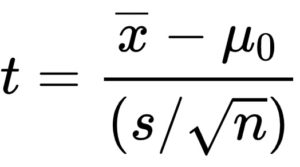In this formula, x bar is again the sample mean, µ is the hypothesized mean, and n is the sample size. The only change is that of ‘s’ which is the sample standard deviation. Moreover, the t critical values are identified from the t-distribution.

Factors such as the sample size, whether the sample distribution is normal, and whether we know the variance, should be considered when deciding whether to use a t-test or a Z confidence interval.

Learn. Apply. Retain.
Your path to achieve medical excellence.
Study for medical school and boards with Lecturio.